Like   Tweet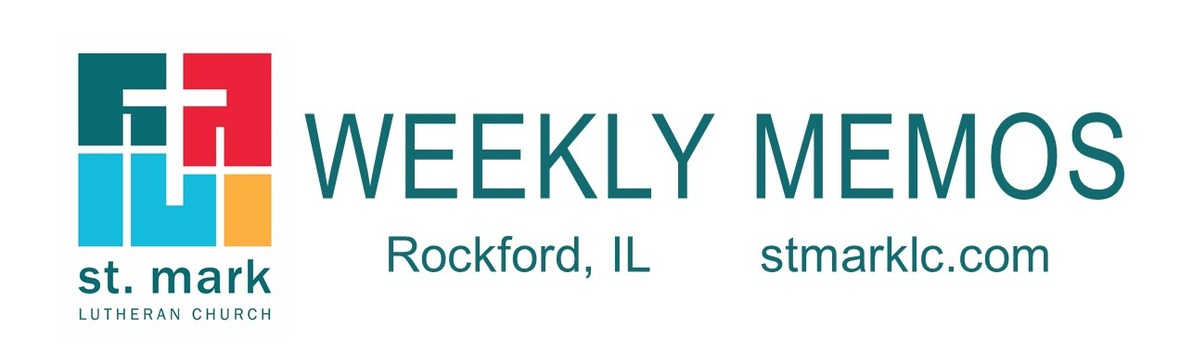table div table+table div table{width:100%;padding:0}table div table+table div table img{width:96.23%;padding:0;float:none}table div table+table div table td{width:100%;padding:0 1.88% 18px}/* styles */## Pastoral Reflection"Will You Walk With Me?"
Pastor Mark Hagen

As you receive this edition of our Memos, Easter is a little over a week away... read more.

 table div table+table+table+table+table div table{width:100%;padding:0}table div table+table+table+table+table div table img{width:96.23%;padding:0;float:none}table div table+table+table+table+table div table td{width:100%;padding:0 1.88% 18px}/* styles */## This Week at St. Mark April 14-21, 2019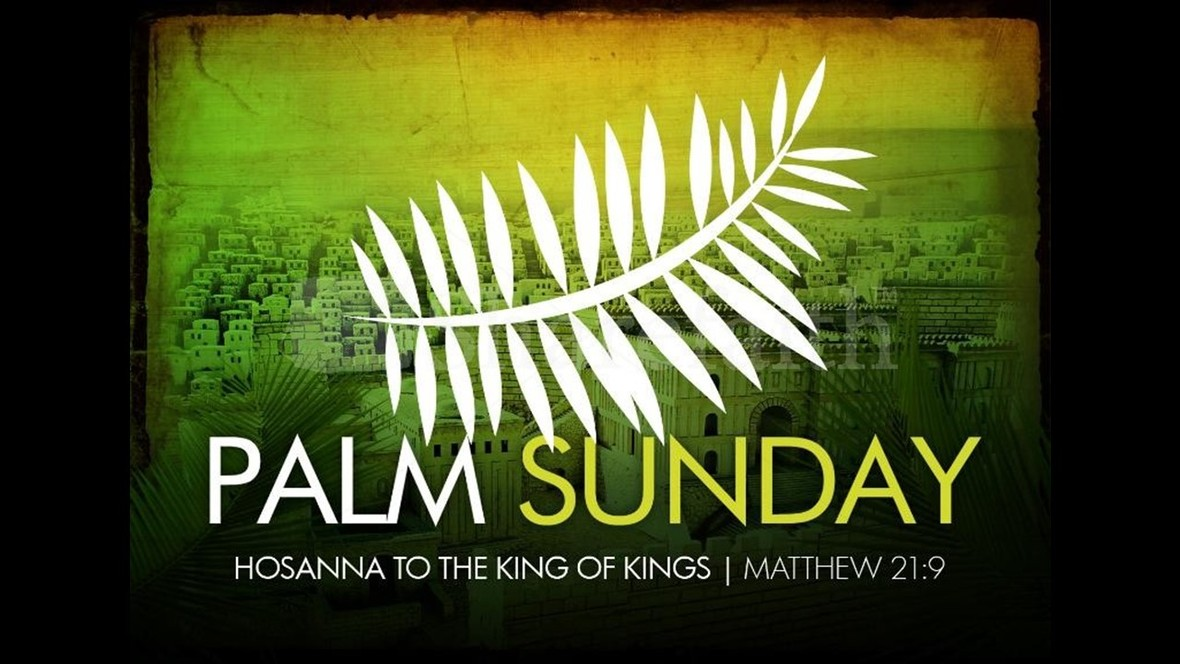## Sunday, April 14 Palm Sunday

8:00 A.M. Worship
9:30 A.M. Worship
9:30 A.M. Sunday School
10:45 A.M. Rev. Robert Connor - Special Presentation on the Cross in the Sanctuary
11:00 A.M. Worship
7:00 P.M. 2nd Sunday at Katie's Cup

## Monday, April 15

9:00 A.M. Classic Chorale Rehearses
6:30 P.M. Blue Lights
6:30 P.M. Emotions Anonymous
7:00 P.M. Theology Pub

## Tuesday, April 16

10:00 A.M. Staff Meeting
11:00 A.M. St. Mark Duplicate Bridge
6:30 P.M. Executive Meeting

## Wednesday, April 17

9:00 A.M. Women's Bible Study
9:00 A.M. Men's Bible Study
9:30 A.M. St. Mark Ringers
12:00 P.M. Primetimers Event in Fellowship Hall
5:30 P.M. Good News Bearers
6:00 P.M. Bible Study
7:00 P.M. Voices of St. Mark

## Thursday, April 18

9:00 A.M. Cherry Valley Bridge Group
12:00 P.M. Maundy Thursday Service
6:30 P.M. Emotions Anonymous
6:30 P.M. Diakonia
6:45 P.M. Maundy Thursday Service
7:00 P.M. Praise Band Rehearses

## Friday, April 19

12:00 P.M. Good Friday Service
6:45 P.M. Good Friday Cantata

## Saturday, April 20

10:00 A.M. Easter Egg Hunt

## Sunday, April 21 Easter

8:00 A.M. Worship
9:30 A.M. Worship
11:00 A.M. Worship

 table div table+table+table+table+table+table+table+table+table+table div table{width:100%;padding:0}table div table+table+table+table+table+table+table+table+table+table div table img{width:96.23%;padding:0;float:none}table div table+table+table+table+table+table+table+table+table+table div table td{width:100%;padding:0 1.88% 18px}/* styles */## News and Events

 table div table+table+table+table+table+table+table+table+table+table+table+table div table{width:100%;padding:0}table div table+table+table+table+table+table+table+table+table+table+table+table div table img{width:96.23%;padding:0;float:none}table div table+table+table+table+table+table+table+table+table+table+table+table div table td{width:100%;padding:0 1.88% 18px}/* styles */Special Presentation on the Cross, April 14

We will begin our Holy Week journey with a special presentation on the cross by Rev. Robert Conner. He will speak at 10:45 in the church nave following the second service on April 14. Rev. Robert Conner is a retired Presbyterian pastor who lives in Rockford. He still does occasional pulpit supply. In 1978, he attended an Arts & Craft class in which he learned to make horse nail crosses (and airplanes with a spark plug body). He makes crosses from old cut nails, in addition to a Faith-Hope-Love wall sculpture. Making the St. Mark cross design was a challenging project. Pastors Mark & Chad both wear this cross throughout the year, as do several church members. Rev. Conner's crosses are also worn by Christians throughout the world. His craft has been the subject of several newspaper articles, including the Rockford Register Star. Rev. Conner will talk about his personal story of cross making, the actual process involved, the meaning of different crosses, and some lessons. Please plan to join us on April 14 for his special presentation at 10:45. Rev. Conner will provide a variety of cross sizes for purchase that day.

 table div table+table+table+table+table+table+table+table+table+table+table+table+table+table div table{width:100%;padding:0}table div table+table+table+table+table+table+table+table+table+table+table+table+table+table div table img{width:96.23%;padding:0;float:none}table div table+table+table+table+table+table+table+table+table+table+table+table+table+table div table td{width:100%;padding:0 1.88% 18px}/* styles */Registration is now open for Vacation Bible School 2019
Volunteer for VBS too at the link below!

To Mars and Beyond: Explore Where God’s Power Can Take You!

Our amazing space adventures will take place from 9 am – noon on June 10-14. VBS is open to elementary-age students and includes daily snacks, crafts, science, games, music, Bible stories, and more! Register in person at St. Mark or online at myvbs.org/stmarklc.
Let’s explore God’s universe together!

 table div table+table+table+table+table+table+table+table+table+table+table+table+table+table+table+table div table{width:100%;padding:0}table div table+table+table+table+table+table+table+table+table+table+table+table+table+table+table+table div table img{width:96.23%;padding:0;float:none}table div table+table+table+table+table+table+table+table+table+table+table+table+table+table+table+table div table td{width:100%;padding:0 1.88% 18px}/* styles */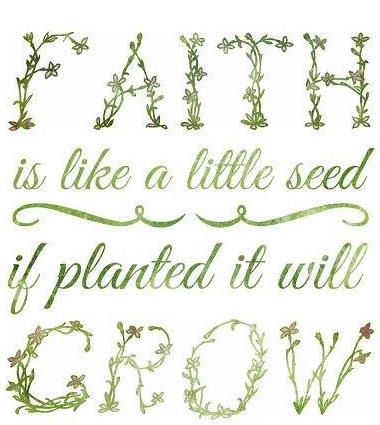Ladies, have you heard? We are having a “spring” event! There are ladies in our life that are amazing! You are amazing! We don’t always get the opportunity to spend enough time with them. If you are available April 27, from 9:00 - noon, we think it will be a wonderful time getting to know the women of St. Mark!

Join with other women of all ages to celebrate spring, after this long winter! Activities will include a speaker, time for relationship building, fun, fellowship and a tasty brunch. There will even be something to “make and take!”

Sign-up in Fellowship Hall April 14th. \$5 suggested donation at the event. Ladies your friends are welcome too!

Childcare will be available if needed. If you need more information please see one of the ladies at the sign-up table in Fellowship Hall, or e-mail melinda@stmarklc.com

“For where two or three are gathered in my name, I am there among them.” Matthew 18:20

 table div table+table+table+table+table+table+table+table+table+table+table+table+table+table+table+table+table+table div table{width:100%;padding:0}table div table+table+table+table+table+table+table+table+table+table+table+table+table+table+table+table+table+table div table img{width:96.23%;padding:0;float:none}table div table+table+table+table+table+table+table+table+table+table+table+table+table+table+table+table+table+table div table td{width:100%;padding:0 1.88% 18px}/* styles */Try-Beta Charitable Foundation, through their member Mark Malenchik, will be selling Beautiful Blooming New Guinea Impatiens Hanging Baskets for \$25 in Fellowship Hall. The sale will occur between services on April 14 and April 28. All baskets will be personally delivered by a member of Try-Beta between May 10 & 11. Cash, Check or Credit Card will be accepted during the sale. Try-Beta Charitable Foundation raises funds with 100% of all funds going to local charities throughout the Rockford Region. Call Mark Malenchik if you are unable to be at church during these times as he will be taking phone orders, 815-519-8501.

 table div table+table+table+table+table+table+table+table+table+table+table+table+table+table+table+table+table+table+table+table div table{width:100%;padding:0}table div table+table+table+table+table+table+table+table+table+table+table+table+table+table+table+table+table+table+table+table div table img{width:96.23%;padding:0;float:none}table div table+table+table+table+table+table+table+table+table+table+table+table+table+table+table+table+table+table+table+table div table td{width:100%;padding:0 1.88% 18px}/* styles */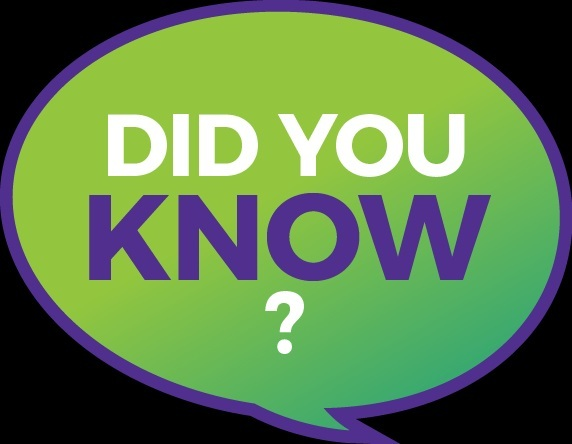Miss a Sunday at St. Mark? Did you know that you can watch the sermons online? Click here to watch.

 table div table+table+table+table+table+table+table+table+table+table+table+table+table+table+table+table+table+table+table+table+table+table div table{width:100%;padding:0}table div table+table+table+table+table+table+table+table+table+table+table+table+table+table+table+table+table+table+table+table+table+table div table img{width:96.23%;padding:0;float:none}table div table+table+table+table+table+table+table+table+table+table+table+table+table+table+table+table+table+table+table+table+table+table div table td{width:100%;padding:0 1.88% 18px}/* styles */Laundry Love Night
On Wednesday, May 15th, St. Mark will sponsor Laundry Love’s monthly open laundry night. In addition to seeking donations the Sunday before, we will also provide the volunteers for the evening. This busy night begins at 5:30 p.m. and ends roughly between 7:30 and 8:00 p.m. after the last client has been served. Volunteers will assist clients with soap and quarters and have plenty of time to talk with those who are there. It is truly a rewarding experience. If you want to participate, please contact Pastor Chad prmckenna@stmarklc.com Space is limited! Laundry Love happens at Charles Street Laundromat (2125 Charles St.) and is a ministry of Rockford Area Lutheran Ministries.

 table div table+table+table+table+table+table+table+table+table+table+table+table+table+table+table+table+table+table+table+table+table+table+table+table div table{width:100%;padding:0}table div table+table+table+table+table+table+table+table+table+table+table+table+table+table+table+table+table+table+table+table+table+table+table+table div table img{width:96.23%;padding:0;float:none}table div table+table+table+table+table+table+table+table+table+table+table+table+table+table+table+table+table+table+table+table+table+table+table+table div table td{width:100%;padding:0 1.88% 18px}/* styles */Happy Birthday, St. Mark!
We give thanks to God for the 58th birthday of our congregation on April 16. We are especially grateful to our charter members and all who have shared in the growth and leadership of St. Mark over these many years!

 table div table+table+table+table+table+table+table+table+table+table+table+table+table+table+table+table+table+table+table+table+table+table+table+table+table+table div table{width:100%;padding:0}table div table+table+table+table+table+table+table+table+table+table+table+table+table+table+table+table+table+table+table+table+table+table+table+table+table+table div table img{width:96.23%;padding:0;float:none}table div table+table+table+table+table+table+table+table+table+table+table+table+table+table+table+table+table+table+table+table+table+table+table+table+table+table div table td{width:100%;padding:0 1.88% 18px}/* styles */St. Mark's Annual Easter Egg Hunt is Saturday, April 20, at 10:00 A.M.

This is always a huge hit with plenty of eggs, games, and fun surprises. That day, we are also collecting old crayons to be re-purposed by Northern Illinois Clean and Beautiful. So, bring the family, an Easter basket, and your old crayons and enjoy this fun morning!

 table div table+table+table+table+table+table+table+table+table+table+table+table+table+table+table+table+table+table+table+table+table+table+table+table+table+table+table+table div table{width:100%;padding:0}table div table+table+table+table+table+table+table+table+table+table+table+table+table+table+table+table+table+table+table+table+table+table+table+table+table+table+table+table div table img{width:96.23%;padding:0;float:none}table div table+table+table+table+table+table+table+table+table+table+table+table+table+table+table+table+table+table+table+table+table+table+table+table+table+table+table+table div table td{width:100%;padding:0 1.88% 18px}/* styles */Family Kite Flying Saturday!
Wind Swept Saturday, May 11, we are hosting a family Kite Flying event. Join us at the Saturn Park for a morning of kite flying and field clean-up. We will celebrate spring and help to make the neighborhood more beautiful! Saturn Park is located at the corner of Garrett Lane and Trainer Road, across from the fire station behind Target. Kites will be provided. Stay tuned for more information.

 table div table+table+table+table+table+table+table+table+table+table+table+table+table+table+table+table+table+table+table+table+table+table+table+table+table+table+table+table+table+table div table{width:100%;padding:0}table div table+table+table+table+table+table+table+table+table+table+table+table+table+table+table+table+table+table+table+table+table+table+table+table+table+table+table+table+table+table div table img{width:96.23%;padding:0;float:none}table div table+table+table+table+table+table+table+table+table+table+table+table+table+table+table+table+table+table+table+table+table+table+table+table+table+table+table+table+table+table div table td{width:100%;padding:0 1.88% 18px}/* styles */I want to say a BIG thank you for all the good wishes on my retirement. I am truly blessed with many beautiful friendships that have come my way over the past 5 years. God’s richest blessings to you, Marsha Scott

 table div table+table+table+table+table+table+table+table+table+table+table+table+table+table+table+table+table+table+table+table+table+table+table+table+table+table+table+table+table+table+table+table div table{width:100%;padding:0}table div table+table+table+table+table+table+table+table+table+table+table+table+table+table+table+table+table+table+table+table+table+table+table+table+table+table+table+table+table+table+table+table div table img{width:96.23%;padding:0;float:none}table div table+table+table+table+table+table+table+table+table+table+table+table+table+table+table+table+table+table+table+table+table+table+table+table+table+table+table+table+table+table+table+table div table td{width:100%;padding:0 1.88% 18px}/* styles */Join Pastor Chad for a pint and great conversation at Carlyle Brewing Co. (215 East State St.) on Monday, April 15 at 7:00 P.M.

 table div table+table+table+table+table+table+table+table+table+table+table+table+table+table+table+table+table+table+table+table+table+table+table+table+table+table+table+table+table+table+table+table+table+table div table{width:100%;padding:0}table div table+table+table+table+table+table+table+table+table+table+table+table+table+table+table+table+table+table+table+table+table+table+table+table+table+table+table+table+table+table+table+table+table+table div table img{width:96.23%;padding:0;float:none}table div table+table+table+table+table+table+table+table+table+table+table+table+table+table+table+table+table+table+table+table+table+table+table+table+table+table+table+table+table+table+table+table+table+table div table td{width:100%;padding:0 1.88% 18px}/* styles */## Primetimers Ministry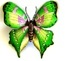On Wednesday, April 17, at Noon the Primetimers are hosting "The Influence of Muslim Traditions and Islamic Inventions In the Arts" by Art Historian Laura Johnson in Fellowship Hall. We will start by serving a pizza lunch at noon. After lunch, Laura will present a slide lecture showing paintings, textiles, leather works, and monuments to demonstrate the influence of the Muslim traditions and Islamic inventions in Florence and Venice during the 15 16th century. She will exhibit how Renaissance artists and architects referred to the teachings of Islamic philosophers and engineers.
You will find this lecture as interesting as last years lecture. I encourage you to bring a friend, invite your neighbor, and please remember to stop by the Primetimers' table and sign up so we may plan accordingly for lunch. We will collect the cost of lunch so bring your wallet.

Save the date, Thursday, May 16. A trip to White Pines Theater. Watch the MEMOS for more details.

 table div table+table+table+table+table+table+table+table+table+table+table+table+table+table+table+table+table+table+table+table+table+table+table+table+table+table+table+table+table+table+table+table+table+table+table+table+table div table{width:100%;padding:0}table div table+table+table+table+table+table+table+table+table+table+table+table+table+table+table+table+table+table+table+table+table+table+table+table+table+table+table+table+table+table+table+table+table+table+table+table+table div table img{width:96.23%;padding:0;float:none}table div table+table+table+table+table+table+table+table+table+table+table+table+table+table+table+table+table+table+table+table+table+table+table+table+table+table+table+table+table+table+table+table+table+table+table+table+table div table td{width:100%;padding:0 1.88% 18px}/* styles */## Youth MinistrySecond Sunday has one more Sunday, April 14, 7:00 P.M. for this school year. We meet at Katie’s Cup. We will follow with a summer campfire event on Sunday, June 9. For the April gathering, all 8th-grade youth are invited to attend. The April theme is thinking of “other’s.” There are games, friends, music and youth-led worship. If you would like a ride please text Melinda Alekna 815-871-0390.

Summer Youth Trip to the Apostle Islands is 24-28. We have a couple of seats left in the van. We hope to sign-up a couple more youth in March. If you have questions or are interested please email or call Melinda Alekna melinda@stmarklc.com or 815-871-0390.

 table div table+table+table+table+table+table+table+table+table+table+table+table+table+table+table+table+table+table+table+table+table+table+table+table+table+table+table+table+table+table+table+table+table+table+table+table+table+table+table+table div table{width:100%;padding:0}table div table+table+table+table+table+table+table+table+table+table+table+table+table+table+table+table+table+table+table+table+table+table+table+table+table+table+table+table+table+table+table+table+table+table+table+table+table+table+table+table div table img{width:96.23%;padding:0;float:none}table div table+table+table+table+table+table+table+table+table+table+table+table+table+table+table+table+table+table+table+table+table+table+table+table+table+table+table+table+table+table+table+table+table+table+table+table+table+table+table+table div table td{width:100%;padding:0 1.88% 18px}/* styles */## Children's Ministry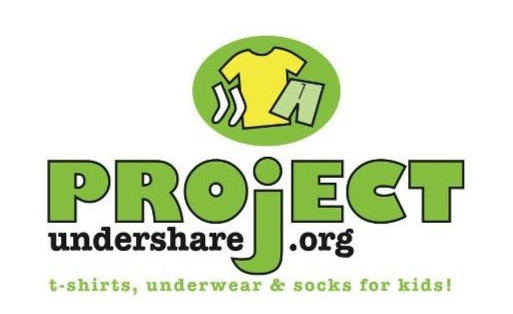Sunday school students will meet downstairs at 9:10, this Sunday, April 14.

Reminder there is no Sunday school on Easter, worship with your families.

Sunday School will be running a Lenten Giving Project, starting next Sunday. For the six weeks of Lent, all of the money collected for Sunday School offering will be donated to Project Undershare, an organization that gives new underwear and socks to children in Rockford who need them.

 table div table+table+table+table+table+table+table+table+table+table+table+table+table+table+table+table+table+table+table+table+table+table+table+table+table+table+table+table+table+table+table+table+table+table+table+table+table+table+table+table+table+table+table div table{width:100%;padding:0}table div table+table+table+table+table+table+table+table+table+table+table+table+table+table+table+table+table+table+table+table+table+table+table+table+table+table+table+table+table+table+table+table+table+table+table+table+table+table+table+table+table+table+table div table img{width:96.23%;padding:0;float:none}table div table+table+table+table+table+table+table+table+table+table+table+table+table+table+table+table+table+table+table+table+table+table+table+table+table+table+table+table+table+table+table+table+table+table+table+table+table+table+table+table+table+table+table div table td{width:100%;padding:0 1.88% 18px}/* styles */## Outreach MinistryThe Outreach Committee is asking members to donate new socks during Lent. The Rockford Public Schools identified the need for socks for the children. Shop all sizes, all colors, and load up the Red Truck in Fellowship Hall! Thanks for your help with this project.

 table div table+table+table+table+table+table+table+table+table+table+table+table+table+table+table+table+table+table+table+table+table+table+table+table+table+table+table+table+table+table+table+table+table+table+table+table+table+table+table+table+table+table+table+table+table+table div table{width:100%;padding:0}table div table+table+table+table+table+table+table+table+table+table+table+table+table+table+table+table+table+table+table+table+table+table+table+table+table+table+table+table+table+table+table+table+table+table+table+table+table+table+table+table+table+table+table+table+table+table div table img{width:96.23%;padding:0;float:none}table div table+table+table+table+table+table+table+table+table+table+table+table+table+table+table+table+table+table+table+table+table+table+table+table+table+table+table+table+table+table+table+table+table+table+table+table+table+table+table+table+table+table+table+table+table+table div table td{width:100%;padding:0 1.88% 18px}/* styles */table div table+table+table+table+table+table+table+table+table+table+table+table+table+table+table+table+table+table+table+table+table+table+table+table+table+table+table+table+table+table+table+table+table+table+table+table+table+table+table+table+table+table+table+table+table+table+table+table div table{width:100%;padding:0}table div table+table+table+table+table+table+table+table+table+table+table+table+table+table+table+table+table+table+table+table+table+table+table+table+table+table+table+table+table+table+table+table+table+table+table+table+table+table+table+table+table+table+table+table+table+table+table+table div table img{width:96.23%;padding:0;float:none}table div table+table+table+table+table+table+table+table+table+table+table+table+table+table+table+table+table+table+table+table+table+table+table+table+table+table+table+table+table+table+table+table+table+table+table+table+table+table+table+table+table+table+table+table+table+table+table+table div table td{width:100%;padding:0 1.88% 18px}/* styles */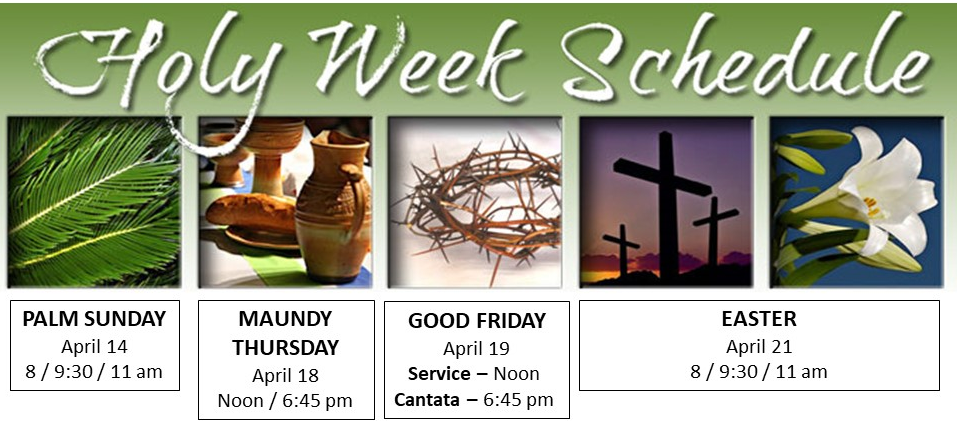table div table+table+table+table+table+table+table+table+table+table+table+table+table+table+table+table+table+table+table+table+table+table+table+table+table+table+table+table+table+table+table+table+table+table+table+table+table+table+table+table+table+table+table+table+table+table+table+table+table+table div table{width:100%;padding:0}table div table+table+table+table+table+table+table+table+table+table+table+table+table+table+table+table+table+table+table+table+table+table+table+table+table+table+table+table+table+table+table+table+table+table+table+table+table+table+table+table+table+table+table+table+table+table+table+table+table+table div table img{width:96.23%;padding:0;float:none}table div table+table+table+table+table+table+table+table+table+table+table+table+table+table+table+table+table+table+table+table+table+table+table+table+table+table+table+table+table+table+table+table+table+table+table+table+table+table+table+table+table+table+table+table+table+table+table+table+table+table div table td{width:100%;padding:0 1.88% 18px}/* styles */table div table+table+table+table+table+table+table+table+table+table+table+table+table+table+table+table+table+table+table+table+table+table+table+table+table+table+table+table+table+table+table+table+table+table+table+table+table+table+table+table+table+table+table+table+table+table+table+table+table+table+table+table div table{width:100%;padding:0}table div table+table+table+table+table+table+table+table+table+table+table+table+table+table+table+table+table+table+table+table+table+table+table+table+table+table+table+table+table+table+table+table+table+table+table+table+table+table+table+table+table+table+table+table+table+table+table+table+table+table+table+table div table img{width:96.23%;padding:0;float:none}table div table+table+table+table+table+table+table+table+table+table+table+table+table+table+table+table+table+table+table+table+table+table+table+table+table+table+table+table+table+table+table+table+table+table+table+table+table+table+table+table+table+table+table+table+table+table+table+table+table+table+table+table div table td{width:100%;padding:0 1.88% 18px}/* styles */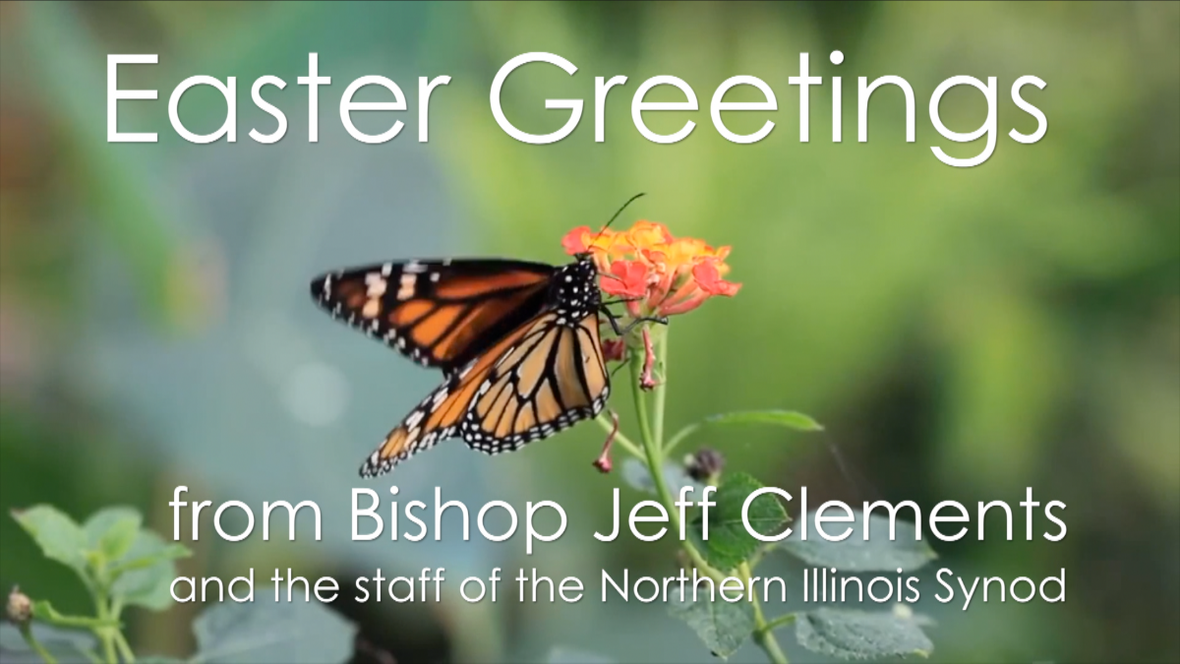table div table+table+table+table+table+table+table+table+table+table+table+table+table+table+table+table+table+table+table+table+table+table+table+table+table+table+table+table+table+table+table+table+table+table+table+table+table+table+table+table+table+table+table+table+table+table+table+table+table+table+table+table+table+table div table{width:100%;padding:0}table div table+table+table+table+table+table+table+table+table+table+table+table+table+table+table+table+table+table+table+table+table+table+table+table+table+table+table+table+table+table+table+table+table+table+table+table+table+table+table+table+table+table+table+table+table+table+table+table+table+table+table+table+table+table div table img{width:96.23%;padding:0;float:none}table div table+table+table+table+table+table+table+table+table+table+table+table+table+table+table+table+table+table+table+table+table+table+table+table+table+table+table+table+table+table+table+table+table+table+table+table+table+table+table+table+table+table+table+table+table+table+table+table+table+table+table+table+table+table div table td{width:100%;padding:0 1.88% 18px}/* styles */## Our St. Mark members:

Rod Bjerke Sr., Kim Edgcomb, David Young, Russ Oellig, Linda Swanson, Pam Cottrill, Keith Haggestad, Naomi Hagen.

## Family and friends of St. Mark members:

Dianne Gill, Denise Nichols, Mary Graham, Doug Nelson, Barbara, Heckart, Tom Wolfe, Kim Menke, Noah and Ellie Adams, Norma Comstock, Scott Jones, Ethan Whitney, Carolyn King, Tom Severson, Katie Butera, Ashton Henley, Nathan Gyllin.

## Those serving in the military and their families:

Bailey Panjkovich, Xander Gehrke, Jim Tammen, Brandon Ballenger, Taylor Eversole, Dean Barron.

 table div table+table+table+table+table+table+table+table+table+table+table+table+table+table+table+table+table+table+table+table+table+table+table+table+table+table+table+table+table+table+table+table+table+table+table+table+table+table+table+table+table+table+table+table+table+table+table+table+table+table+table+table+table+table+table+table+table div table{width:100%;padding:0}table div table+table+table+table+table+table+table+table+table+table+table+table+table+table+table+table+table+table+table+table+table+table+table+table+table+table+table+table+table+table+table+table+table+table+table+table+table+table+table+table+table+table+table+table+table+table+table+table+table+table+table+table+table+table+table+table+table div table img{width:96.23%;padding:0;float:none}table div table+table+table+table+table+table+table+table+table+table+table+table+table+table+table+table+table+table+table+table+table+table+table+table+table+table+table+table+table+table+table+table+table+table+table+table+table+table+table+table+table+table+table+table+table+table+table+table+table+table+table+table+table+table+table+table+table div table td{width:100%;padding:0 1.88% 18px}/* styles *//* styles */ Office Hours Monday - Friday, 8:00 A.M. - 4:00 P.M. Telephone: 815-398-3557 Publications St. Mark Memos, the weekly e-newsletter, is distributed each Thursday. The deadline for submission is Monday by 4:00 P.M. Please click to send submissions. Sermons Online Miss a Sunday at St. Mark? Please visit our website and watch the sermons online. Please click to watch.